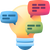# States of matter ( Gaseous State)

How would you define universal gas constant R

This post was part of TyroCity discussion forumDiscuss User 2 • Edited

To understand the concept, let’s start by first taking ideal gas law (PV = nRT) and rearranging it for R. It gives us the following equation:

R = PV/nT

With that equation now known, we can calculate R for any gas situation, we’ll obviously get the sae number each time though. Let’s try solving it for a gas situation that we’re aware of in Avogadro’s Law. This states that 1 mole of an ideal gas at a standard pressure and temperature (or 1 atmosphere and 273 K) takes up 22.4 ltr. of space.

With these values known, if we were to put it in the above equation, we’ll have the following:

P = 1 atmosphere
V = 22.4 liters
n = 1 mol
T = 273 K

Putting that in equation, we’ll have this:

R = 1 x 22.4 / 1 x 273
i.e. R = 22.4 / 273
i.e. R = 0.0821 L atm per mol K (rounded figure)

So, if you know Avogadro’s Law, there’s no need to really memorize R, you can simply calculate it as well.

Please do note that this constant number (0.0821) is different depending on which units of pressure and volume you use. So, if you always use liters, atmospheres, moles, and Kelvin, the 0.0821 will always work. However, deepening on the situation and need you may have to convert units like Celsius to Kelvin or torr to atmospheres and so on.

The gas constant or R is an important constant in the ideal gas law.

This post was part of TyroCity discussion forum